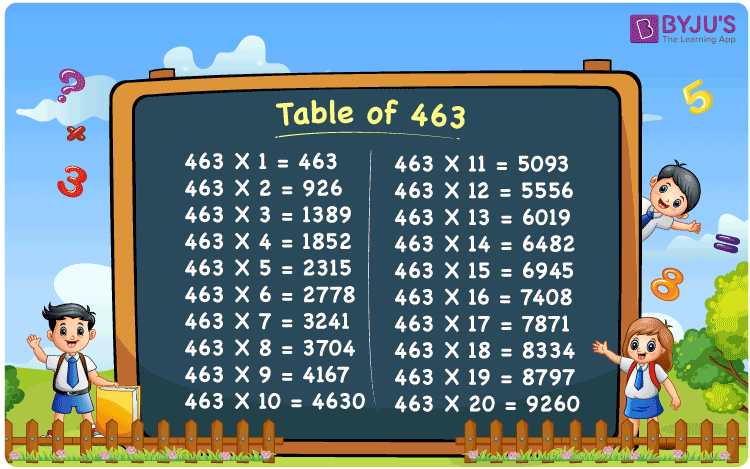Checkout JEE MAINS 2022 Question Paper Analysis : Checkout JEE MAINS 2022 Question Paper Analysis :

# Table of 463

The Table of 463 is made available at BYJU’S in PDF format, according to the convenience of the students. The multiplication tables can be either viewed online or downloaded while solving problems, based on the multiples of 463. For a good hold on the tables, students are recommended to revise the multiplication table of 463 on a daily basis. It also aims at improving the problem-solving abilities of the school going children beginning from their junior classes itself.

## Table of 463 Chart## What is the 463 Times Table?

The multiplication table of 463 should be given more priority from the primary grades so that solving problems based on the fundamental concepts in Maths would be easy. The concept of repetitive addition, which is used while multiplying a certain number with another, is explained in simple language. Chart of the same is attached below for the reference of the students.

 463 × 1 = 463 463 463 × 2 = 926 463 + 463 = 926 463 × 3 = 1389 463 + 463 + 463 = 1389 463 × 4 = 1852 463 + 463 + 463 + 463 = 1852 463 × 5 = 2315 463 + 463 + 463 + 463 + 463 = 2315 463 × 6 = 2778 463 + 463 + 463 + 463 + 463 + 463 = 2778 463 × 7 = 3241 463 + 463 + 463 + 463 + 463 + 463 + 463 = 3241 463 × 8 = 3704 463 + 463 + 463 + 463 + 463 + 463 + 463 + 463 = 3704 463 × 9 = 4167 463 + 463 + 463 + 463 + 463 + 463 + 463 + 463 + 463 = 4167 463 × 10 = 4630 463 + 463 + 463 + 463 + 463 + 463 + 463 + 463 + 463 + 463 = 4630

## Multiplication Table of 463

The Table of 463 has to be memorized thoroughly by the students who wish to build their career in the Engineering stream. The reason behind this is, multiplication mainly discusses the importance of numbers in our daily lives. So one who masters these concepts will be able to answer most of the complex questions that would be asked in their final exams.

 463 × 1 = 463 463 × 2 = 926 463 × 3 = 1389 463 × 4 = 1852 463 × 5 = 2315 463 × 6 = 2778 463 × 7 = 3241 463 × 8 = 3704 463 × 9 = 4167 463 × 10 = 4630 463 × 11 = 5093 463 × 12 = 5556 463 × 13 = 6019 463 × 14 = 6482 463 × 15 = 6945 463 × 16 = 7408 463 × 17 = 7871 463 × 18 = 8334 463 × 19 = 8797 463 × 20 = 9260

## Solved Example on the Table of 463

Q.1: 3 faculty conducted classes in a day. How many faculty will conduct the class in 463 days?

Solution: Given,

Number of faculty who conduct the classes in a day = 3

In 463 days number of faculty who will be conducting the classes = 463 x 3

= 1389

## Frequently Asked Questions on the Table of 463

### What is 463 multiplied by 13?

From the table of 463, 463 multiplied by 13 is 463 x 13 = 6019.

### How many times should 463 be multiplied to get 8797?

Using the table of 463, 463 x 19 = 8797. Hence, 463 should be multiplied by 19 times to get 8797.

### Find the value of 463 times 10 plus 7 minus 15.

In the table of 463, 463 times 10 plus 7 minus 15 can be written as 463 x (10 + 7 – 15) = 463 x 2 = 926